# Find angle B in the trigonometry problem

Gold Member
Homework Statement:
Kindly see the attached problem and find angle ##B##
Relevant Equations:
sine and cosine rule
Now i was just doing some random study and i saw this problem on the internet. The problem was to find angle ##B##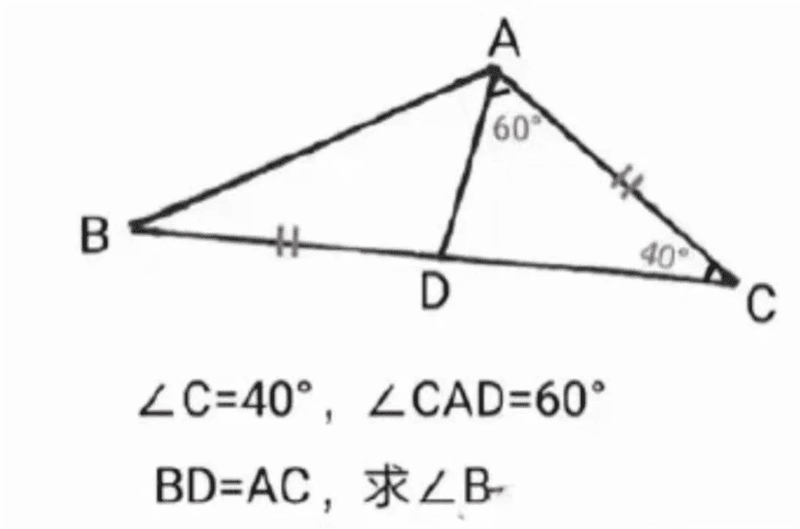now there were several responses, let me screen shot one that i think was a bit straightforward...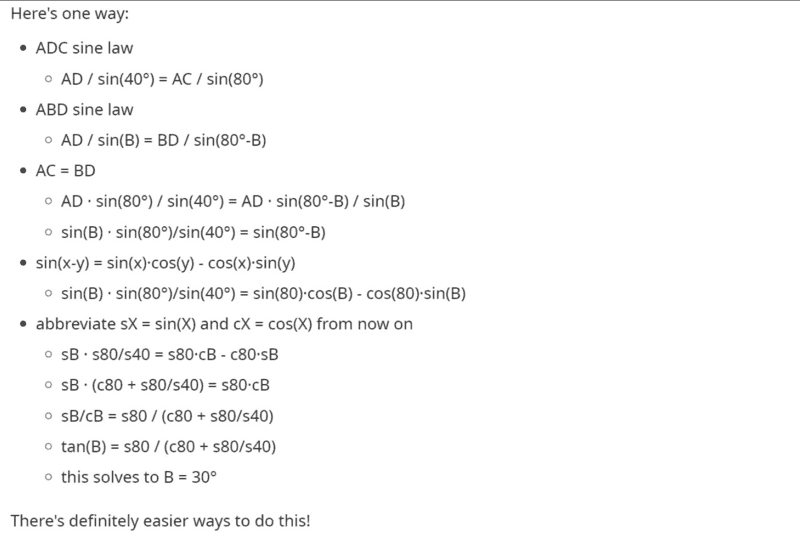my question is, "is there a much simpler way to find the angle ##B##"?

phinds
Gold Member
my question is, "is there a much simpler way to find the angle ##B##"?
What have you tried so far?

•berkeman
Gold Member
What have you tried so far?
This is another solution from the internet: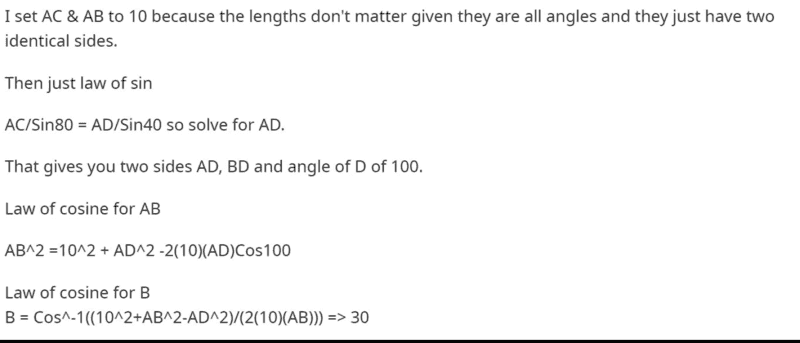With a slight variation from the attached solution i came up with this:
Following the assumption that ##AC=AB=10cm## then it follows that,
##AB^2##=##10^2+6.527^2-(2×10×6.527×cos 100)##
##AB=12.85cm##
##\frac {12.85}{sin100}##=##\frac {6.527}{sin B}##
##sin B=0.5002210##
##B= sin^{-1}(0.5002210)=30^0##

Last edited:
neilparker62
Homework Helper
Just for a challenge: prove ##\tan\hat B=\frac{1}{\sqrt{3}}##. No calculator.

Gold Member
Just for a challenge: prove ##\tan\hat B=\frac{1}{\sqrt{3}}##. No calculator.
you must be kidding...one may use the equilateral triangle to show this....have a perpendiculor bisector...
....ok let me check it out neilneilparker62
Homework Helper
You cannot use your destination in your journey - you can't assume 30 degrees at B before you have proven the ratio (no calculator allowed).

Gold Member
Find my attempt...i cannot seem to find the ratio that you talked about...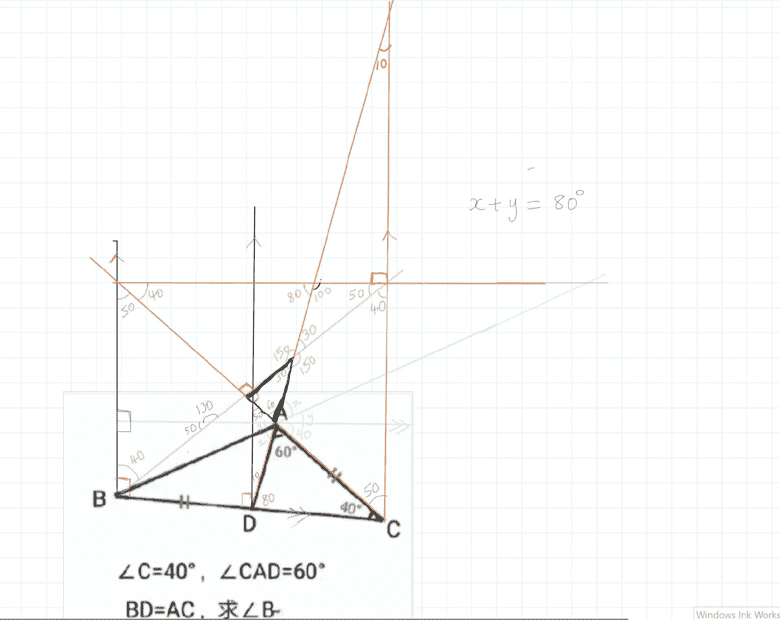neilparker62
Homework Helper
You have diagonals marked perpendicular in the middle of your construction above. This would be the case if the corresponding quadrilateral is a square. But that's not the case otherwise the diagonals would bisect the corner angles 45/45. I tried geometric constructions and got nowhere so I reverted to trig manipulations to prove the ratio ##\tan\hat B=\frac{1}{\sqrt{3}}## and hence that ##\hat B=30^{\circ}##.

Gold Member
You have diagonals marked perpendicular in the middle of your construction above. This would be the case if the corresponding quadrilateral is a square. But that's not the case otherwise the diagonals would bisect the corner angles 45/45. I tried geometric constructions and got nowhere so I reverted to trig manipulations to prove the ratio ##\tan\hat B=\frac{1}{\sqrt{3}}## and hence that ##\hat B=30^{\circ}##.
Phew it has boggled my mind ...almost 2 hours just looking at this...neilparker62
Homework Helper
You will need to make use of the "tan rule". See following diagram: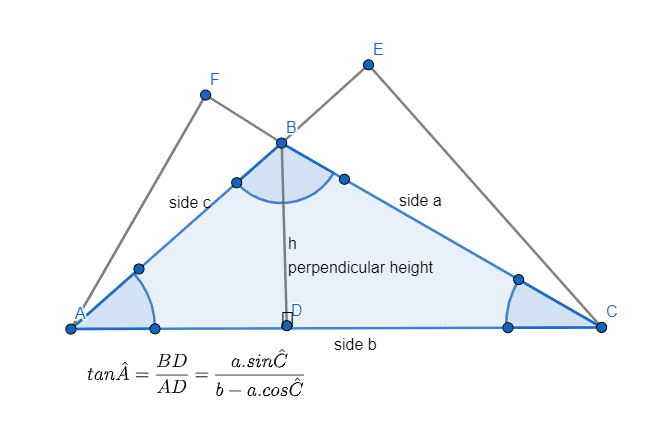Gold Member
Thanks, kindly confirm the dimensions in your diagram, is your ##BD= AC## as indicated in the problem? I do not think so...and further angle ##C= 40^0##, i do not see how one can use thinking of right angle (in your case the tangent approach) at this points as the angles in that triangle ##ADC##are , ##60^0, 80^0## and ##40^0## respectively (see my post ##7##)...how did you come up with the common perpendiculor ##BD##?

Last edited:
neilparker62
Homework Helper
It's not at all the same diagram as in the problem - it's a diagram intended to show you how the tan rule works

Gold Member
I understand your diagram, it's straightforward...the problem is I do not see how to relate it with the original problem with reasons given... i. e no common perpendicular that will realize two right angle triangles to work with.

neilparker62
Homework Helper
To get BC (in terms of x) you will first need DC (also in terms of x). You can use the sine rule for that.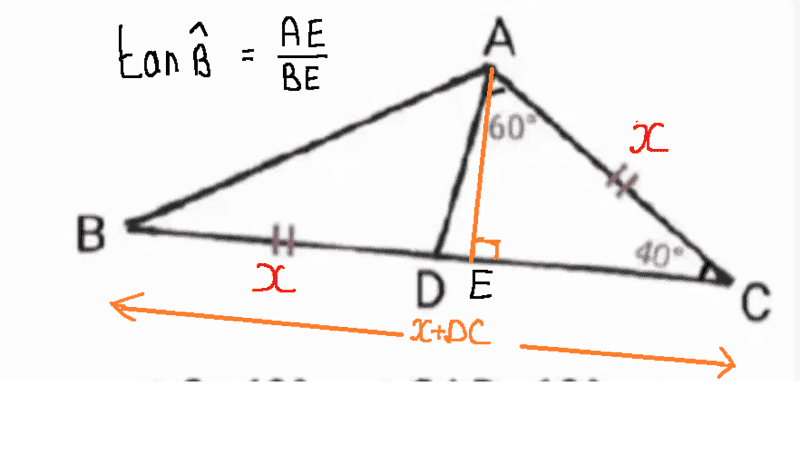•chwala
Gold Member
I had seen that...we will need to find length of ##DE## for tangent rule to apply...and I do not think that's possible. I don't think finding ##BC## or ##DC## or ##BD## would help...
How to show proof of your post ##4## without Calculator would be interesting...

neilparker62
Homework Helper
Give some careful thought to what goes where when the tan rule formula is applied to your problem. Alternatively you can actually get an expression for DE in terms of x. In that case you would first need to find AD.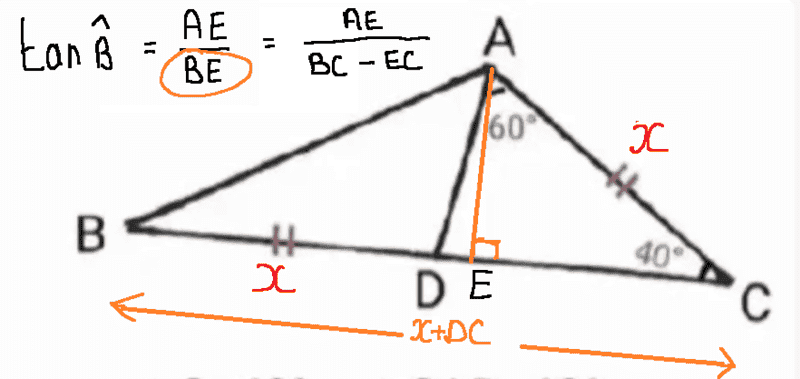#### Attachments

Last edited:
•chwala
Gold Member
Okay, i managed to do this,
##tan 80##=## \frac {AE}{ED}##
and we know that;
##AE=x sin 40^0## therefore,
##DE##=## \frac {x sin 40^0⋅cos 80^0}{sin 80^0}##
##tan B##=## \frac {x⋅sin 40^0⋅sin 80^0}{x⋅sin 80^0+x⋅sin 40^0⋅cos 80^0}##...................................(1)
##[cos 40^0-cos 120^0]=2sin 80^0sin 40^0##→##0.5[cos 40^0-cos 120^0]=sin 80^0sin 40^0##
##[sin 120^0-sin 40^0]= 2 cos 80^0 sin40^0##→##0.5[sin 120^0-sin 40^0]= cos 80^0 sin40^0##
##tan B##=## \frac {-0.5cos 120^0+0.5cos40^0}{sin 80^0+0.5sin 120^0-0.5sin40^0}##
##tan B##=## \frac {0.25+0.5 \frac {EC}{x}}{\frac {AE}{AD}+0.25 \sqrt 3-0.5 \frac {AE}{x}}##

alternatively,
##cos 40^0##=## \frac {sin 80^0}{2 sin 40^0}##
therefore from (1) above we shall have,
##tan B##=## \frac {sin 80^0}{ 2 cos 40^0 +cos 80^0}## on dividing each term by ##sin 80^0##
##tan B##=## \frac {1}{\frac {1}{sin 40^0}+\frac {cos 80^0}{sin 80^0}}##=## \frac {1}{\frac {1}{sin 40^0}+\frac {sin 10^0}{sin 80^0}}##
Neil am stuck here mateLast edited:
neilparker62
Homework Helper
##tan B##=## \frac {sin 80^0}{ 2 cos 40^0 +cos 80^0}## on dividing each term by ##sin 80^0##

Neil am stuck here mate80 = 120 - 40

Gold Member
ok by substituting ##80=120-40## in the equation ##tan B##=## \frac {sin 80^0}{ 2 cos 40^0 +cos 80^0}## and after simplification, i end up with,
##tan B##=## \frac {\sqrt 3 cos 40^0 + sin 40^0}{ 3 cos 40^0+\sqrt 3 sin 40^0}##=## \frac {\sqrt 3 + tan 40^0}{ 3 +\sqrt 3 tan40^0}##=## \frac {1}{ \sqrt 3}##⋅## \frac {\sqrt 3 + tan 40^0}{ \sqrt 3 +tan40^0}##=## \frac {1}{ \sqrt 3}## Bingo!Last edited:
neilparker62
Homework Helper
ok by substituting ##80=120-40## in the equation ##tan B##=## \frac {sin 80^0}{ 2 cos 40^0 +cos 80^0}## and after simplification, i end up with,
##tan B##=## \frac {\sqrt 3 cos 40^0 + sin 40^0}{ 3 cos 40^0+\sqrt 3 sin 40^0}##=## \frac {\sqrt 3 + tan 40^0}{ 3 +\sqrt 3 tan40^0}##=## \frac {1}{ \sqrt 3}##⋅## \frac {\sqrt 3 + tan 40^0}{ \sqrt 3 +tan40^0}##=## \frac {1}{ \sqrt 3}## Bingo!Mission accomplished!

Last edited:
•Gold Member
Homework Helper
Gold Member
2020 Award
This problem is interesting. By law of sines, etc. I got
## \tan{B}=\frac{\sin(40)}{1+\frac{\sin(60)}{\sin(80)}-\cos(40)} ##.
I set this equal to ## \frac{1}{\sqrt{3}} ##, and was able to show consistency with a lot of algebra.
Using ## \cos(120)=-\frac{1}{2} ## and ## \cos(3 \theta)=4 \cos^3(\theta)-3 \cos(\theta) ##, we have
## \cos^3(40)=(6 \cos(40)-1)/8 ##, and I was able to simplify things, but it took a lot of work. It also involved grouping ## \sin(40)=\sqrt{1-\cos^2(40)} ## terms together, and squaring both sides. I managed to get it to two expressions with ## \cos^2(40),\cos(40) ##, and constant terms, and I was able to get both expressions to agree.
Note: ## \sin(60)=\sqrt{3}/2 ##, and ## \sin(80)=2 \sin(40) \cos(40) ##.

Last edited:
•chwala
neilparker62
Homework Helper
This problem is interesting. By law of sines, etc. I got
## \tan{B}=\frac{\sin(40)}{1+\frac{\sin(60)}{\sin(80)}-\cos(40)} ##.
You can get from here to the expression in post #17 by writing sin(60) as sin(100-40) - noting that sin(100)=sin(80) and cos(100)=-cos(80). Begin by multiplying through by sin(80).

•Homework Helper
Gold Member
2020 Award
You can get from here to the expression in post #17 by writing sin(60) as sin(100-40) - noting that sin(100)=sin(80) and cos(100)=-cos(80). Begin by multiplying through by sin(80).
This one is interesting in that there still doesn't seem to be a very simple way to get 30 degrees for B. Without a calculator to give us a clue, like in post 3, we wouldn't have guessed that the expressions are indeed that ## \tan{B}=\frac{1}{\sqrt{3}} ##. (My first instincts were that my expression in post 22 might give something close to 30 degrees, but it wouldn't be exactly 30 degrees).

neilparker62
Homework Helper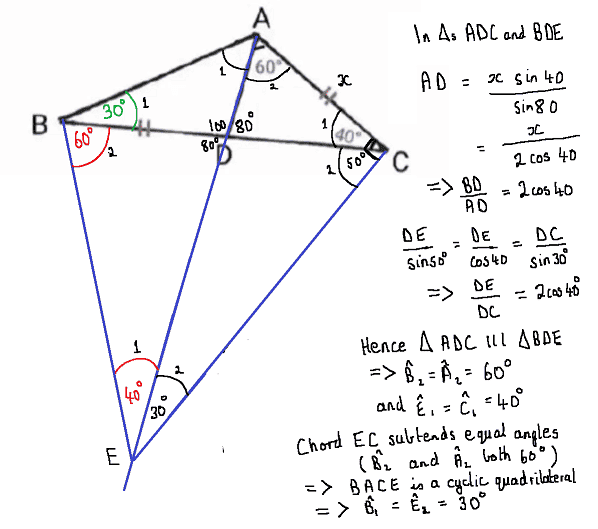•